# Pressure & Its Measurement - 2

## 10 Questions MCQ Test Topicwise Question Bank for GATE Mechanical Engineering | Pressure & Its Measurement - 2

Description
This mock test of Pressure & Its Measurement - 2 for Mechanical Engineering helps you for every Mechanical Engineering entrance exam. This contains 10 Multiple Choice Questions for Mechanical Engineering Pressure & Its Measurement - 2 (mcq) to study with solutions a complete question bank. The solved questions answers in this Pressure & Its Measurement - 2 quiz give you a good mix of easy questions and tough questions. Mechanical Engineering students definitely take this Pressure & Its Measurement - 2 exercise for a better result in the exam. You can find other Pressure & Its Measurement - 2 extra questions, long questions & short questions for Mechanical Engineering on EduRev as well by searching above.
QUESTION: 1

### What should be the property of good manometer fluid

Solution:

Manometric fluid should have low vapour pressure and high density to have less height of piezometric tube.
Since Mercury have both the property hence this is the reason, Mercury used as Manometric fluid.

QUESTION: 2

### Fluid is a substance which offers no resistance to change of

Solution:

A fluid does not offer any resistance to change of shape and deformed under the action of small force.

QUESTION: 3

### When can a piezometer be not used for pressure measurement in pipes

Solution:

Limitations of Piezometer:
1. Piezometers cannot be used to measure pressure which are considerably excess of atmospheric pressure use of very long glass tube would be unsafe. It being both Fragile and unmanageable.
2. Gas pressure cannot be measured as gas does not form any free surface with atmosphere.
3. Measurement of negative pressure is not possible due to flow of atmospheric air into the container through the tube.

QUESTION: 4

The instrument preferred in the measurement of highly fluctuating velocities in air flows in

Solution:

Hot wire anemometer is used in the measurement of highly fluctuating velocities in air flow.

QUESTION: 5

How is the difference of pressure head h measured by mercury oil differential manometer expressed
Sg and So are the specific gravities of mercury and oil respectively

Solution: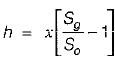Where
Sg = Specific gravity of Mercury
So = Specific gravity of Oil
Here, Sg > So

QUESTION: 6

Differential manometer is used to measure

Solution:
QUESTION: 7

In order to increase sensitivity of U-tube manometer, one leg is usually inclined by angle θ, sensitivity of inclined tube to sensitivity of U-tube equal to

Solution: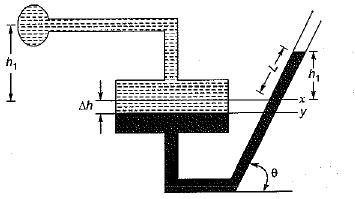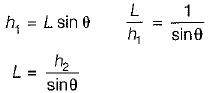QUESTION: 8

The specific weight of ocean water changes according to the equation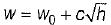where w0 = specific weight of ocean water at the free surface, then pressure as a function of depth is

Solution: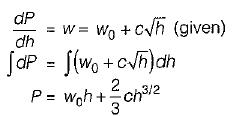QUESTION: 9

The standard atmospheric pressure is 762 mm of Hg. At a specific location, the barometer reads 700 mm of Hg. At this place, what does at absolute pressure of 380 mm of Hg corresponds to?

Solution:

Reference is always taken from atmospheric pressure which is indicated by Barometer. Barometer reads the local atmospheric pressure.
So pressure = 700-380 = 320 mm of Hg vacuum.

QUESTION: 10

In figure if the pressure of gas in bulb A is 50 cm Hg vacuum and Patm = 76 cm Hg, the height of column H is equal to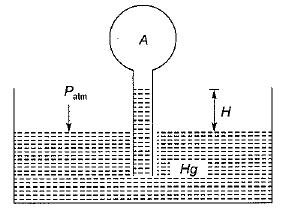Solution:

In a fluid at rest, pressure through out the any horizontal line is same.

We have, PA = 50 cm Hg vaccum=-ρhgg50

and, Patm hggH = PA for gauge pressure Patm=0
0 - ρhggH = -ρhgg50 ...[-ve sign for vaccum Pressure]

H=50 cm